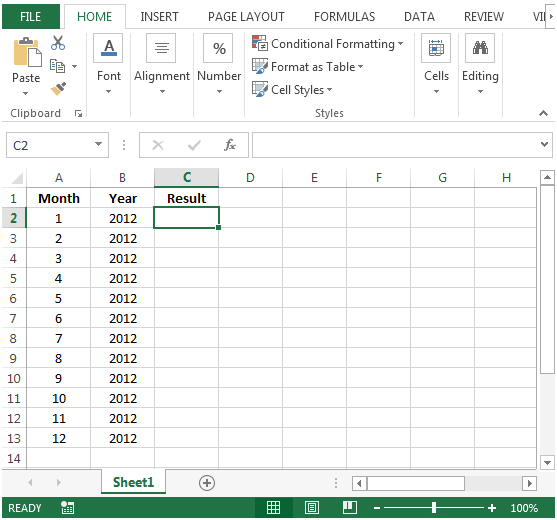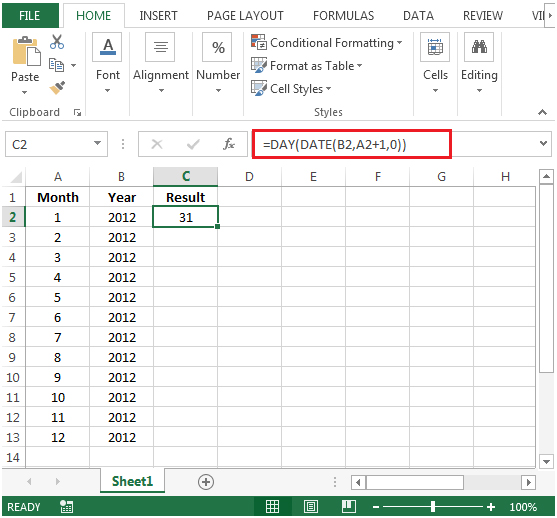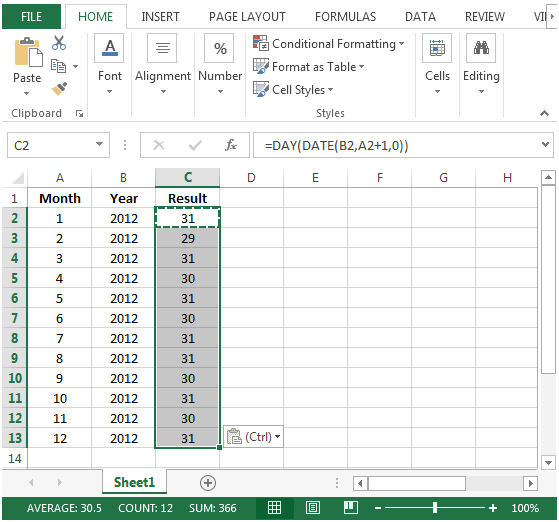# Finding the Number of Days in a Given Month in Microsoft Excel 2010

To find the number of days in a given month, we can use the combination of DATE & DAY functions to get the output.

DATE: Returns the number that represents the date in Microsoft Excel date-time code.

Syntax: =DATE(year,month,day)

year: The year argument can include one to four digits. By default,Excel uses the 1900 date system.

month:It is the second argument that represents the month of the year from 1 to 12 (January to December)

day: It is the third argument that represents the day of the month from 1 to 31.

DAY: Returns the day of the month, a number from 1 to 31

Syntax: =DAY(serial_number)

serial_number: Itrefers to the day of the month that you are trying to find.

Let us take an example

Column A contains month number & column B contains year.In column C, we need a formula to calculate the number of days in each of the month listed in column A, for the year specified in column B.• Write the formula in cell C2.
• =DAY(DATE(B2,A2+1,0))
• Press Enter on your keyboard.• Copying down the formula from cell C2 to range C3:C13, we will get the desired result.Terms and Conditions of use

The applications/code on this site are distributed as is and without warranties or liability. In no event shall the owner of the copyrights, or the authors of the applications/code be liable for any loss of profit, any problems or any damage resulting from the use or evaluation of the applications/code.